# Black Scholes ( Black 76 ) Formula in Endur with Delayed Payment (from Exercise Date)

#### by Israr Ahmed

In this Article we look at the academic definition of how Black Scholes is used to value options on forwards/futures – in the context of delayed payment from exercise date. We then look at how this is implemented in Endur. We also have a version of the Endur BS Formula in an online Spreadsheet format.

Black 76

Black Scholes is used to price Spot prices. To model Future/Forward prices we assumes Futures are like a stock paying dividend q equal to r. The standard Black-Scholes with dividend collapses into: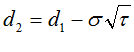## Black 76 extension: Delayed Payment Date

In reality the actual Payment Dategenerally occurs later than the Expiry Date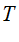ie.

We can think of the derivative becoming a cash position (equal to it’s value) at expiration. Such a cash position does not accrue interest until payment date. Therefore this type of contract must be further discounted to take into account the delay in payment.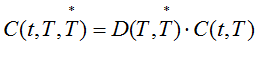=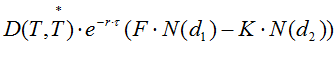(A)

whereis the discount from the Payment Date back to the Exercise Date ienow substituting this into (A) we can also re-write(B)

(A) and (B) are equivalent

whereis the zero rate at Expiry Date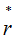is the zero rate at Payment Date## OpenLinks B-S ( Black 76 ) Model with Delayed Payment Date

There are a number of modifications to consider:

• OpenLink sets q (the dividend) to = r (risk free rate) so that the B-S Model essentially becomes the Black 76 Model
• OpenLink actually calculates the B-S (ie Black 76) value as at the Payment Date using the modified Black 76 extension shown above.
•= time to Expiration (with CC Zero Rate r )
•= time to Payment (with CC Zero Rate)

Given that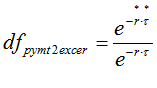thenThe formula can then be written in two different way – essentially exactly the same – but Formula 2 is more explicit.

Formula 1 ( as implemented by OpenLink )Formula 2 (more explicit)where we have the usual , time t (exercise date ) calculated;Note: OpenLink gets the value for{ie ‘R’ in model input screen} by recalculating the CC Zero Rate using Act/365 rather than what is on the LIBOR curve setting